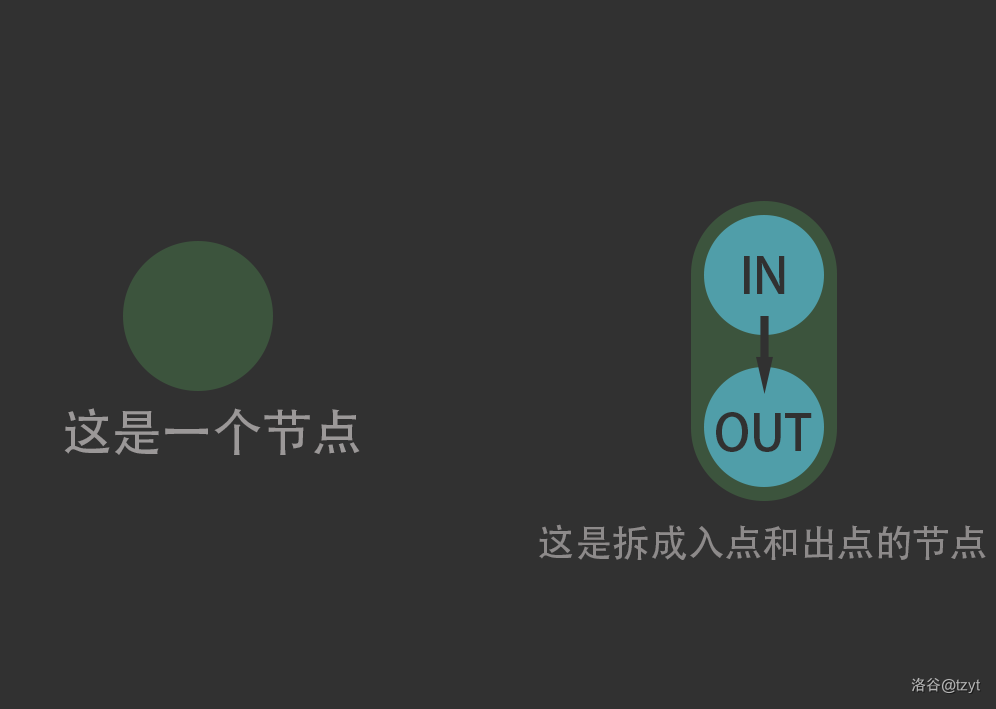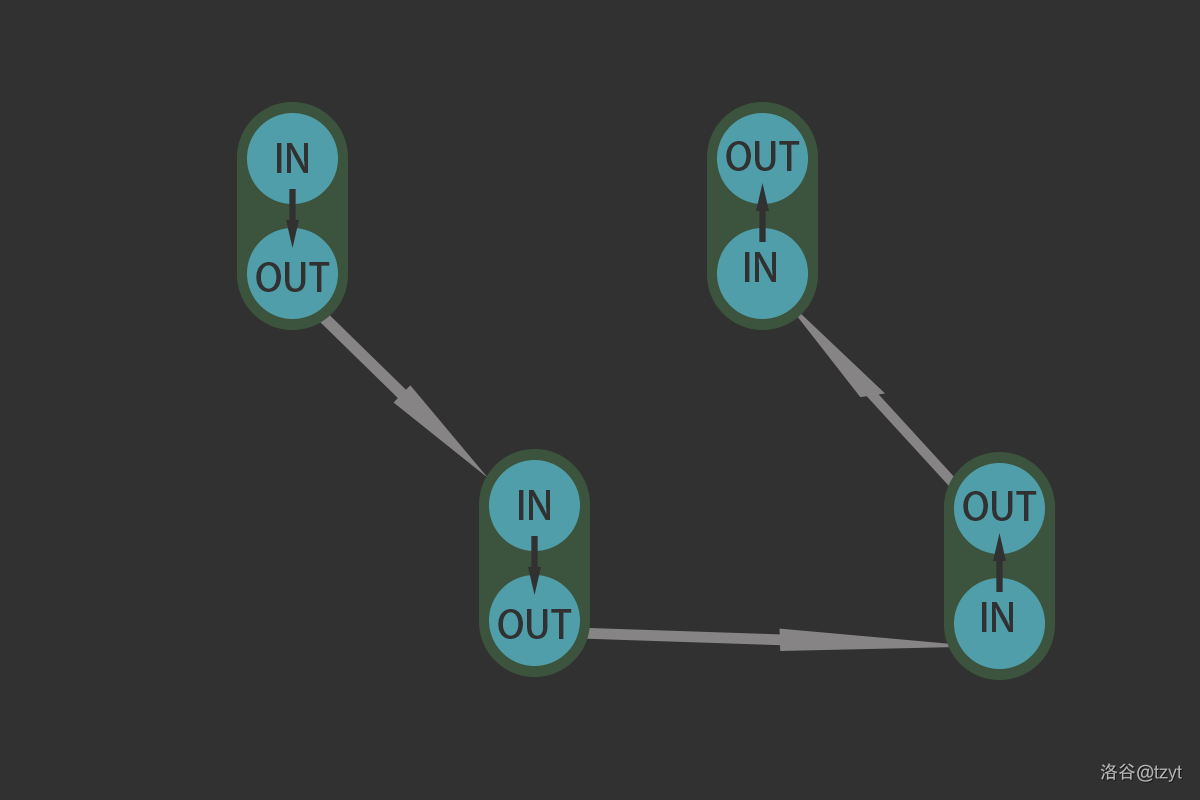# 2：分析和建模

【不熟悉最大流算法的同学可以先做一下模板题】

P1345 奶牛的电信Telecowmunication## 建图步骤总结：

1. 把每个节点拆成入点和出点，中间连一条内部边
2. 对于能删除的点，内部边的容量设置成$1$
3. 对于不能删除的点，内部边容量设置成 $INF$
4. 不能删除的边包括：
1. 源点的内部边
2. 汇点的内部边
3. 连接每个节点的边
4. 关键点的内部边
5. 源点设置成$1$号节点，汇点连接所有的关键点

# 4.代码实现及细节

#include <bits/stdc++.h>
using namespace std;
const int MAXM = 100000;
const int INF = 0x3f3f3f3f;
struct node
{
int to, mflow, rev; //to连接的下个节点的编号
//mflow(maxflow)记录当前边的容量
//rev(reverse)记录当前边的反向边的下标
};
int p, c, n, s, t;
vector<node> edge[MAXM];
int g_farm[MAXM];                            //完好的农场（关键点）
int layer[MAXM];                             //每个节点的层数
node assign_node(int to, int mflow, int rev) //赋值函数
{
node temp;
temp.to = to, temp.mflow = mflow, temp.rev = rev;
return temp;
}

void add_edge(int from, int to, int mflow) //加边
{
edge[from].push_back(assign_node(to, mflow, edge[to].size()));   //不需要-1是因为edge[to]还没有push过 from这个节点
edge[to].push_back(assign_node(from, 0, edge[from].size() - 1)); //-1是因为vector的下标是从0开始的，而.size()会返回元素的数量
}

namespace dinic
{
bool layering() //分层
{
bool vis[MAXM];
memset(vis, false, sizeof(vis));
memset(layer, 0, sizeof(layer));
queue<int> q;
vis[s] = true;
layer[s] = 1;
q.push(s);
while (!q.empty())
{
int cur = q.front();
q.pop();
for (auto nex : edge[cur]) //c++11的新特性，意思是用nex遍历edge[cur]中的所有元素
{
if (nex.mflow > 0 && vis[nex.to] == false)
{
layer[nex.to] = layer[cur] + 1;
q.push(nex.to);
vis[nex.to] = true;
}
}
}
return (layer[t] != 0); //返回分层操作是否成功（是否能从源点到达汇点）
}

int find_aug_path(int cur, int cur_flow) //寻找增广路
{
if (cur == t)
{
return cur_flow;
}
int ans = 0;
for (int i = 0; i < int(edge[cur].size()); i++)
{
if (edge[cur][i].mflow > 0 && layer[edge[cur][i].to] == layer[cur] + 1)
{
int nex_flow = find_aug_path(edge[cur][i].to, min(cur_flow, edge[cur][i].mflow));
edge[cur][i].mflow -= nex_flow;                            //正向边
edge[edge[cur][i].to][edge[cur][i].rev].mflow += nex_flow; //反向边
cur_flow -= nex_flow;
ans += nex_flow;
if (cur_flow <= 0) //如果当前的容量已经不够了，就直接返回来节省时间
{
return ans;
}
}
}
return ans;
}

int find_maxflow()
{
int ans = 0;
while (layering())
{
ans += find_aug_path(s, INF);
}
return ans;
}
}

void input_creat() //输入和建图
{
scanf("%d%d%d", &p, &c, &n);
s = 0, t = 2 * p + 1;
add_edge(1, 1 + p, INF); //源点的入点和出点设置成INF
for (int i = 1; i <= c; i++)
{
int from, to;
scanf("%d%d", &from, &to);
add_edge(from + p, to, INF); //from的出点和to的入点相连
add_edge(to + p, from, INF); //to的出点和from的入点相连
}
for (int i = 1; i <= n; i++)
{ //n是不能割的点
int point;
scanf("%d", &point);
add_edge(point + p, t, INF);     //把所有关键点连接到汇点
add_edge(point, point + p, INF); //所有关键点的内部边的容量都要设成INF
g_farm[point] = 1;               //标记关键点
}
for (int i = 2; i <= p; i++)
{
if (!g_farm[i])
{
add_edge(i, i + p, 1); //除了关键点的其他点可以删，所以内部边的容量设成1
}
}
}

main()
{
input_creat();
printf("%d", dinic::find_maxflow());
system("pause");
}